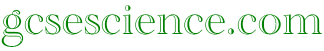GCSE Physics 2015
Revision Quizzes

Below is a full list of all
the
physics quizzes in alphabetical order.

Acceleration - Speed - Velocity

Alpha Particles - Beta Particles - Gamma Rays

Big bang - Red-Shift - Universe

Cancer - Smoke Alarm - Thickness Control - Tracer

Carbon Dating - Detection - Half-Life

Circuit Breaker - Electromagnetism - Motor

Comets - The Moon - Satellites

Current - Power - Voltage

Detection of Radioactivity (see Carbon Dating)

Diode - LDR - Resistance - Thermistor

Earthquakes - Plate Tectonics

Efficiency - Insulation

Electromagnetic Waves

Electromagnetism (see Circuit Breaker)

Electrostatic Charge

Energy - Kinetic - Potential - Power

Energy Resources - Power Stations

Fission - Nuclear Power - Rutherford

Force - Gravity - Mass - Weight

Friction - Motion - Stopping Distance

Galaxies - Stars

Gamma Rays (see Alpha Particles)

Generator - Transformer

Gravity (see Force)

Half-Life (see Carbon Dating)

Insulation (see Efficiency)

Kinetic Energy (see Energy)

LDR  (see Diode)

Lenses

Light

Longitudinal - Transverse - Waves

Magnets

Mass (see Force)

Momentum

Moon (see Comets)

Motion (see Friction)

Motor (see Circuit Breaker)

Nuclear Power (see Fission)

Nucleus - Isotope

Planets - Solar System

Plate Tectonics (see Earthquakes)

Potential Energy (see Energy)

Power - Electrical (see Current)

Power - Energy (see Energy)

Power - Nuclear (see Fission)

Red-Shift (see Big Bang)

Resistance - Resistor (see Diode)

Rutherford (see Fission)

Satellites (see Comets)

Smoke Alarm (see Cancer)

Solar System (see Planets)

Sound

Speed (see Acceleration)

Stars (see Galaxies)

Stopping Distance (see Friction)

Tracer - Thickness Control (see Cancer)

Transformer (see Generator)

Transverse (see Longitudinal)

Velocity (see Acceleration)

Voltage (see Current)

Universe (see Big Bang)

Waves (see Longitudinal)

Weight (see Force)

Home          GCSE Physics          Index          Chemistry Quiz List